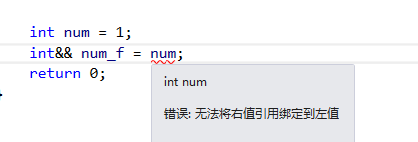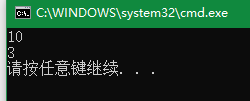﻿ 左值与右值引用 详解

说明

int a=10;            //a 是左值

double b=1.3;		 //b 是左值

int & Ta=a;	    	//引用左值   故 是一个左值引用

double & Tb=b;	    //引用左值 故是一个左值引用

‘a‘  是常量  故为右值
3    是常量  故为右值
1+3     运算会将 1+3 的结果保存至 寄存器 此时并不在特定的内存位置 故 该值为右值 是临时变量

{
return 0;
}   		//该函数返回的值并不会保存在内存中 仅出现在临时量中  语句执行完毕后 该临时量被销毁

char && a="a";	        //常量
int && b=1+3; 	        //临时变量
int&& c= add();   	    //函数引用

左值引用实例

#include<iostream>
using namespace std;
int main(void)
{
int a=10;                //左值
int & In_a=a;            //左值引用
cout<<"Old:"<<In_a<<endl;

In_a=2;	          	     //改变别名
cout<<"New:"<<In_a<<endl;

return 0;
}右值引用实例

#include<iostream>
using namespace std;

{
return 0;
}										//返回常量 0

int fun(int&& f)						//形参为右值引用
{
cout << f << endl;
return 1;
}										//返回常量 1
int main(void)
{
‘a‘;								//常量 ‘a‘
3;									//常量  3
1 + 3;								//寄存器量 1+3结果 4

//使用右值引用

char && Char_a = ‘a‘;
cout << "‘a‘:" << Char_a << endl;

int && Int_a = 3;
cout << "3:" << Int_a << endl;

int && Int_b = 1 + 3;
cout << "1+3:" << Int_b << endl;

int &&Int_c = add();
cout << "add()return 0:" << Int_c << endl;

int && Int_d = fun(3);    //输出3
cout << "fun() 3:" << Int_d << endl;

return 0；
}#include<iostream>

using namespace std;

template<typename T> 					//函数模板
void fun(T&& f)							//兼容 左值引用 和右值引用
{
cout << f << endl;
}

int main(void)
{
int a = 10;
fun(a);
fun(3);	   //兼容 左值引用 和右值引用

return 0;
}(0)
(0)© 2014 xscp18.com 版权所有 京ICP备13008772号-2